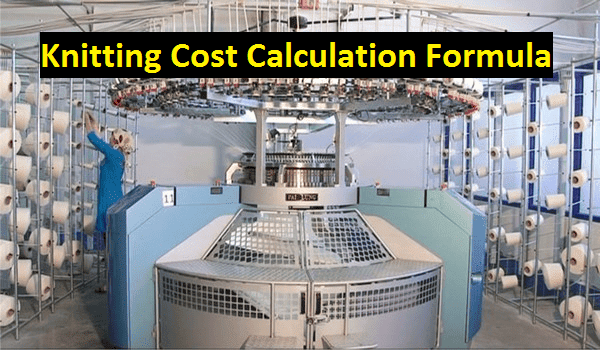# Apparel Costing

Solution of textile and apparel calculation

## Important Formulas of Knitting Cost Calculation in Textile

Calculation of Knitting Cost:
Before starting the knitting cost calculation, you have to know some major factors of cost calculation for it. This factor includes knitting cost elements, knitting cost calculation formulas and clear ideas about approximate knitting cost in the surrounding factory. This article has presented all the matters which have needed to fulfill a knitting cost calculation in textile industry.Fig: Knitting cost calculation formula in textile
Elements of Knitting Cost:
The cost elements of the knitting process are as follows:

D.M= Direct material i.e. yarn.
D.L= Direct labor i.e. operators and helpers in production, inspection.
D.E= Direct expenses

Knitting Cost Factors:
The cost of knitting process varies from company to company due to a lot of causes or reasons. There are some key factors which are deeply influenced in knitting cost have presented in the below:
1. Types of fabric i.e. single jersey, rib etc.
2. Types of design i.e. auto stripes, jacquard etc.
3. Types of raw material i.e. spandex yarn etc.
4. Types of machine i.e. conventional, modern etc.
5. Production capacity for the factory or company.
Knitting Cost Calculation Formulas:
The below formulas or laws have to follow during knitting cost calculation in textile sector.

1. Fabric Manufacturing and Selling Cost Calculation:
If the knit fabric manufacturing factory produces knit fabric and sell it to the outside customers then the below formula have to follow for calculating knit fabric cost.

Knit fabric cost per kg,

Direct material (D.M) + Direct labor (D.L) + Direct expenses (D.E) + Overhead (OH)
= ……………………………………..............................................…………………………………
Total knit fabric production

2. Knit Fabric Manufacturing Cost calculation:
If the knit fabric manufacturing factory does job work for knitting process only then the formula to calculate the knitting cost is as in the following:

Knitting cost per kg,

Direct labor (D.L) + Direct expenses (D.E) + Overhead (OH)
= …………………………………………………………………………
Total fabric production